不同声带长度的声门管内流场数值模拟Numerical Simulation of the Glottal Flow Field with Different Lengths of Vocal Cord

• 全文下载: PDF(1872KB)    PP.139-148   DOI: 10.12677/IJM.2019.82016
• 下载量: 165  浏览量: 471   国家自然科学基金支持

In order to investigate the distribution of pressure field in the glottal duct with different vocal cord lengths, three different glottal models were established by using finite element analysis method. Five different glottal diameters (0.01 cm, 0.02 cm, 0.04 cm, 0.08 cm, 0.16 cm) and three kinds of subglottal pressure (500 pa, 1000 pa, 1500 pa) were set up in the model respectively. The pressure distribution is obtained by numerical simulation of the flow field by ANSYS Fluent. The experimental results show that the longer glottal length will reduce the pressure drop at the en-trance of the glottal, which helps make it easier to create vibration of the vocal folds and reduces the phonation threshold pressure, and provide reference for further study of the vocal mechanism of human vocal cords and the repair of damaged vocal cords.

1. 引言

2. 声带建模与边界条件

2.1. 声带几何模型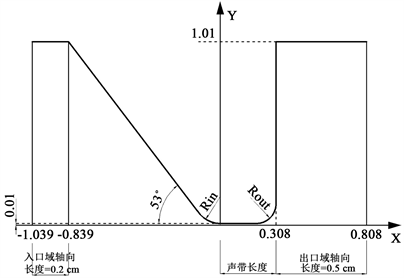Figure 1. Vocal cord simulation model

2.2. 网格划分与边界条件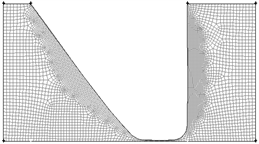Figure 2. Vocal cord mesh model

3. 声门管流场分析基本理论

3.1. 连续性方程

$\frac{\partial \rho }{\partial t}+\frac{\partial \left(\rho {v}_{x}\right)}{\partial x}+\frac{\partial \left(\rho {v}_{y}\right)}{\partial y}+\frac{\partial \left(\rho {v}_{z}\right)}{\partial z}=0$ (1)

$\frac{\partial {v}_{x}}{\partial x}+\frac{\partial {v}_{y}}{\partial y}+\frac{\partial {v}_{z}}{\partial z}=0$ (2)

3.2. Navier-Stokes方程

$\begin{array}{l}\rho \left(\frac{\partial {v}_{x}}{\partial t}+{v}_{x}\frac{\partial {v}_{x}}{\partial x}+{v}_{y}\frac{\partial {v}_{x}}{\partial y}+{v}_{z}\frac{\partial {v}_{x}}{\partial z}\right)=-\frac{\partial p}{\partial x}+\mu \left(\frac{{\partial }^{2}{v}_{x}}{\partial {x}^{2}}+\frac{{\partial }^{2}{v}_{x}}{\partial {y}^{2}}+\frac{{\partial }^{2}{v}_{x}}{\partial {z}^{2}}\right)\\ \rho \left(\frac{\partial {v}_{y}}{\partial t}+{v}_{x}\frac{\partial {v}_{y}}{\partial x}+{v}_{y}\frac{\partial {v}_{y}}{\partial y}+{v}_{z}\frac{\partial {v}_{y}}{\partial z}\right)=-\frac{\partial p}{\partial y}+\mu \left(\frac{{\partial }^{2}{v}_{y}}{\partial {x}^{2}}+\frac{{\partial }^{2}{v}_{y}}{\partial {y}^{2}}+\frac{{\partial }^{2}{v}_{y}}{\partial {z}^{2}}\right)\\ \rho \left(\frac{\partial {v}_{z}}{\partial t}+{v}_{x}\frac{\partial {v}_{z}}{\partial x}+{v}_{y}\frac{\partial {v}_{z}}{\partial y}+{v}_{z}\frac{\partial {v}_{z}}{\partial z}\right)=-\frac{\partial p}{\partial z}+\mu \left(\frac{{\partial }^{2}{v}_{z}}{\partial {x}^{2}}+\frac{{\partial }^{2}{v}_{z}}{\partial {y}^{2}}+\frac{{\partial }^{2}{v}_{z}}{\partial {z}^{2}}\right)\end{array}$ (3)

3.3. SST k-ω模型方程

$\begin{array}{l}\frac{\partial }{\partial t}\left(\rho {u}_{i}\right)+\frac{\partial }{\partial {x}_{i}}\left(\rho {u}_{i}{u}_{j}\right)=-\frac{\partial p}{\partial {x}_{i}}+\frac{\partial }{\partial {x}_{j}}\left(\Gamma \frac{\partial {u}_{i}}{\partial {x}_{j}}\right)+{S}_{i},\left(i=1,2,3\right)\\ \frac{\partial }{\partial t}\left(\rho k\right)+\frac{\partial }{\partial {x}_{i}}\left(\rho k{u}_{j}\right)=\frac{\partial }{\partial {x}_{j}}\left({\Gamma }_{k}\frac{\partial k}{\partial {x}_{j}}\right)+{\stackrel{˜}{G}}_{k}-{Y}_{k}+{S}_{k}\\ \frac{\partial }{\partial t}\left(\rho \omega \right)+\frac{\partial }{\partial {x}_{j}}\left(\rho \omega {u}_{j}\right)=\frac{\partial }{\partial {x}_{j}}\left({\Gamma }_{\omega }\frac{\partial \omega }{\partial {x}_{j}}\right)+{G}_{\omega }-{Y}_{\omega }+{D}_{\omega }+{S}_{\omega }\end{array}$ (4)

4. 实验结果与分析Table 1. Vocal cord length of 0.108 cmTable 2. Vocal cord length of 0.308 cm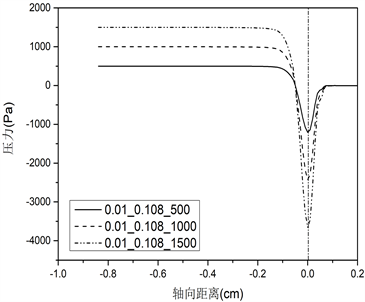(i)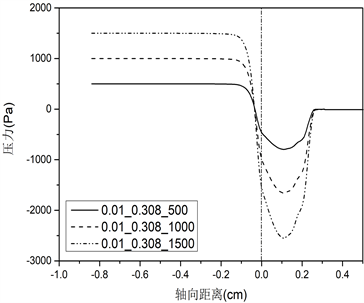(ii)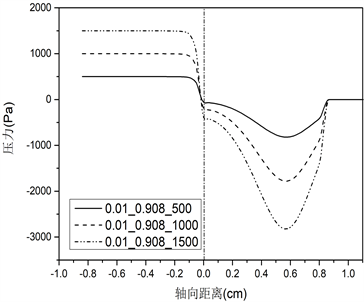(iii) (a)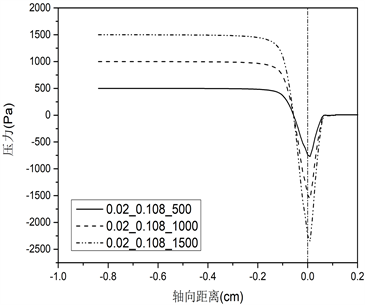(i)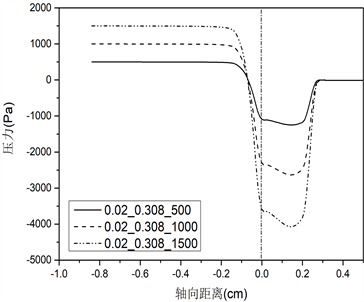(ii)(iii) (b)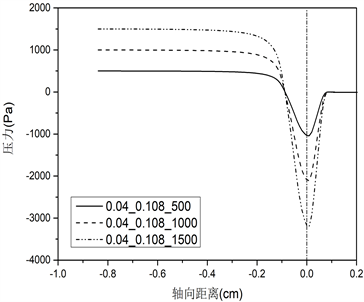(i)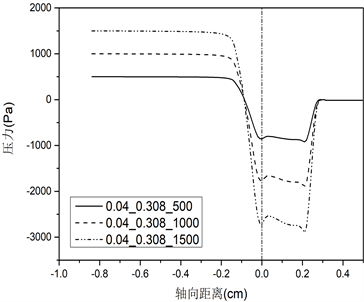(ii)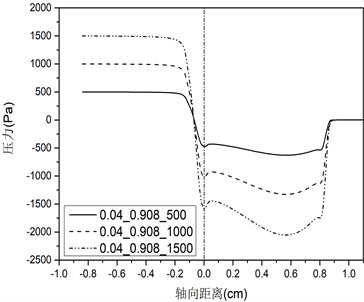(iii) (c)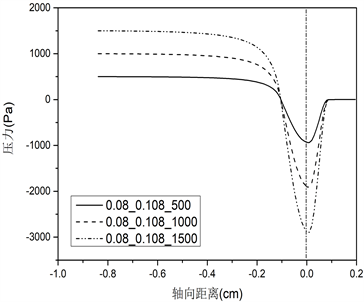(i)(ii)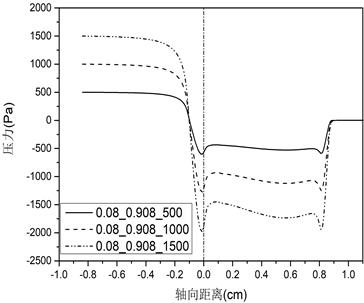(iii) (d)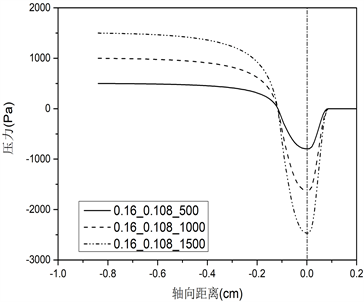(i)(ii)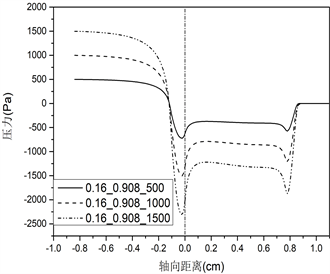(iii)(e)

Figure 3. Surface pressure distribution of vocal cords corresponding to different vocal cords lengths. (a) 0.01 cm and (b) 0.02 cm, (i) 0.108 cm, (ii) 0.308 cm, (iii) 0.908 cm; (c) 0.04 cm and (d) 0.08 cm, (i) 0.108 cm, (ii) 0.308 cm, (iii) 0.908 cm; (e) 0.16 cm, (i) 0.108 cm, (ii) 0.308 cm, (iii) 0.908 cmTable 3. Vocal cord length of 0.908 cm

5. 结论

  Titze, I.R. (1980) Comments on the Myoelastic-Aerodynamic Theory of Phonation. Journal of Speech & Hearing Re-search, 23, 495-510. https://doi.org/10.1044/jshr.2303.495  Ishizaka, K. and Flanagan, J.L. (1972) Synthesis of Voiced Sounds from a Two-Mass Model of the Vocal Cords. The Bell System Technical Journal, 51, 1233-1268. https://doi.org/10.1002/j.1538-7305.1972.tb02651.x  Titze, I.R. (1988) The Physics of Small-Amplitude Os-cillation of the Vocal Folds. The Journal of the Acoustical Society of America, 83, 1536. https://doi.org/10.1121/1.395910  Steinecke, I. and Herzel, H. (1995) Bifurcations in an Asymmetric Vo-cal-Fold Model. The Journal of the Acoustical Society of America, 97, 1874-1884. https://doi.org/10.1121/1.412061  Story, B.H. and Titze, I.R. (1995) Voice Simulation with a Body-Cover Model of the Vocal Folds. The Journal of the Acoustical Society of America, 97, 1249. https://doi.org/10.1121/1.412234  Alipour, F., Fan, C. and Scherer, R.C. (1996) A Numerical Simulation of Laryngeal Flow in a Forced-Oscillation Glottal Model. Computer Speech and Language, 10, 75-93. https://doi.org/10.1006/csla.1996.0005  Alipour, F. (2004) Flow Separation in a Computational Oscillating Vocal Model. The Journal of the Acoustical Society of America, 116, 1710. https://doi.org/10.1121/1.1779274  Zheng, X., Mittal, R. and Bielamowicz, S. (2011) A Computational Study of Asymmetric Glottal Jet Deflection during Phonation. The Journal of the Acoustical Society of America, 129, 2133-2143. https://doi.org/10.1121/1.3544490  Fulcher, L.P., Scherer, R.C. and Powell, T. (2011) Pressure Distributions in a Static Physical Model of the Uniform Glottis: Entrance and Exit Coefficients. The Journal of the Acoustical Society of America, 129, 1548-1553.https://doi.org/10.1121/1.3514424  Scherer, R.C., Shinwari, D., Witt, K.J.D., et al. (2001) Intraglottal Pressure Profiles for a Symmetric and Oblique Glottis with a Divergence Angle of 10 Degrees. The Journal of the Acoustical Society of America, 109, 1616-1630.https://doi.org/10.1121/1.1333420  Fulcher, L.P., Scherer, R.C., Witt, K.J.D., et al. (2010) Pressure Distributions in a Static Physical Model of the Hemilarynx: Measurements and Computations. Journal of Voice, 24, 2-20. https://doi.org/10.1016/j.jvoice.2008.02.005  Scherer, R.C., Witt, K.J.D. and Kucinschi, B.R. (2001) The Effect of Exit Radii on Intraglottal Pressure Distributions in the Convergent Glottis. Journal of the Acoustical Society of America, 110, 2267-2269. https://doi.org/10.1121/1.1408255  Li, S., Scherer, R.C., Wan, M.-X., Wang, S.-P. and Wu, H.-H. (2006) Numerical Study of the Effects of Inferior and Superior Vocal Fold Surface Angles on Vocal Fold Pressure Distributions. Journal of the Acoustical Society of America, 119, 3003-3010. https://doi.org/10.1121/1.2186548  Li, S., Wan, M.-X. and Wang, S.-P. (2008) The Effects of the False Vocal Fold Gaps on Intralaryngeal Pressure Distributions and Their Effects on Phonation. Science in China Series C: Life Sciences, 51, 1045-1051. https://doi.org/10.1007/s11427-008-0128-3  Titze, I.R. (1992) Phonation Threshold Pressure: A Missing Link in Glottal Aerodynamics. The Journal of the Acoustical Society of America, 91, 2926. https://doi.org/10.1121/1.402928  Suh, J. and Frankel, S.H. (2008) Comparing Turbulence Models for Flow through a Rigid Glottal Model. The Journal of the Acoustical Society of America, 123, 1237. https://doi.org/10.1121/1.2836783